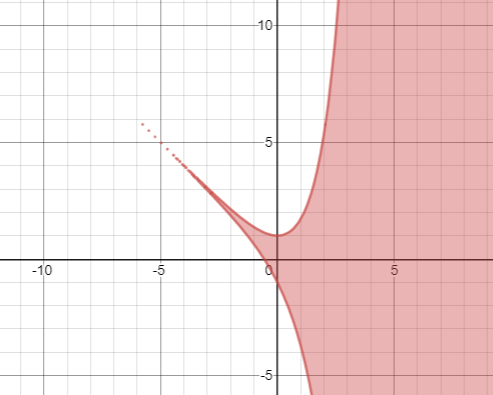# The set of all points ( x , y ) which satisfy the given condition by sketching it .### Single Variable Calculus: Concepts...

4th Edition
James Stewart
Publisher: Cengage Learning
ISBN: 9781337687805### Single Variable Calculus: Concepts...

4th Edition
James Stewart
Publisher: Cengage Learning
ISBN: 9781337687805

#### Solutions

Chapter 4, Problem 14P
To determine

## The set of all points (x,y) which satisfy the given condition by sketching it .

Expert Solution

### Explanation of Solution

Given information:

The condition is,

|x+y|ex

Calculations:

Here the given condition is,

|x+y|ex

Now we draw the graph using graphing software and show all set of values. The shaded region shows the set off values.### Have a homework question?

Subscribe to bartleby learn! Ask subject matter experts 30 homework questions each month. Plus, you’ll have access to millions of step-by-step textbook answers!